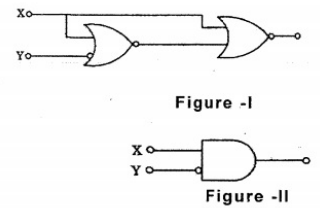Related Articles
ISRO | ISRO CS 2008 | Question 25
• Difficulty Level : Hard
• Last Updated : 20 Nov, 2018

The logic operations of two combinational circuits in Figure-I and Figure-II are(A) entirely different
(B) identical
(C) complementary
(D) dual

Explanation: The Two functions are entirely different as:

Figure 1: The logic gates derive the following function:

```F1 = ((X + Y')' + X)'
= ((X + Y')')'. X'
= (X + Y'). X'
= XX' + X'Y'
= X'Y'```

Figure 2: It is simple AND gate which has 1 input already complimented.

`F2 = XY'`

So, these two functions are entirely different.

Quiz of this Question

My Personal Notes arrow_drop_up